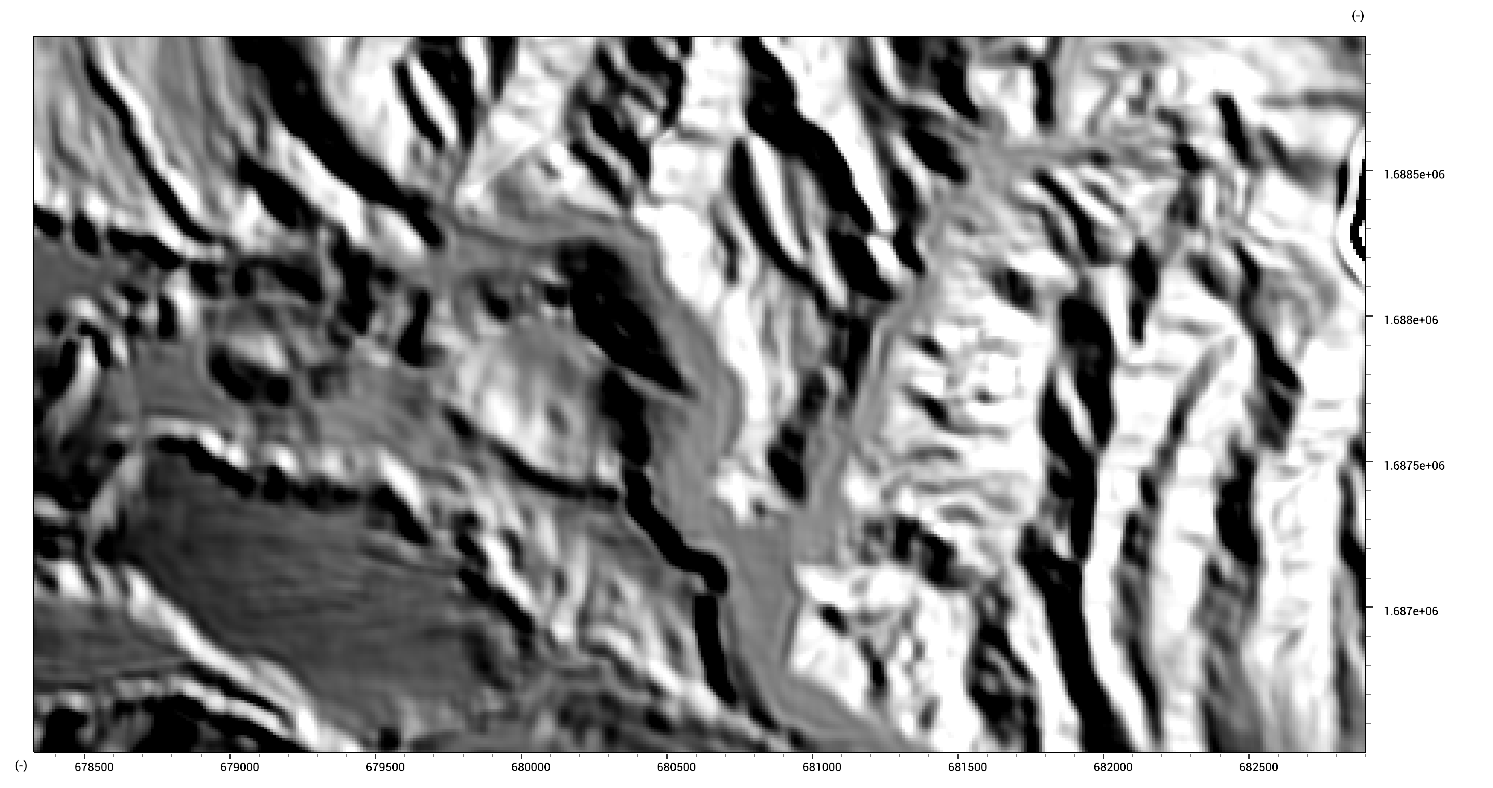LISEM Classic

LISEM

# Filters

The window-filters provide operations to a specified window. The WindowAverage function effectively blurs and smoothenes the data.

dem_lidar_wa.map = WindowAverage(dem_lidar.map,100);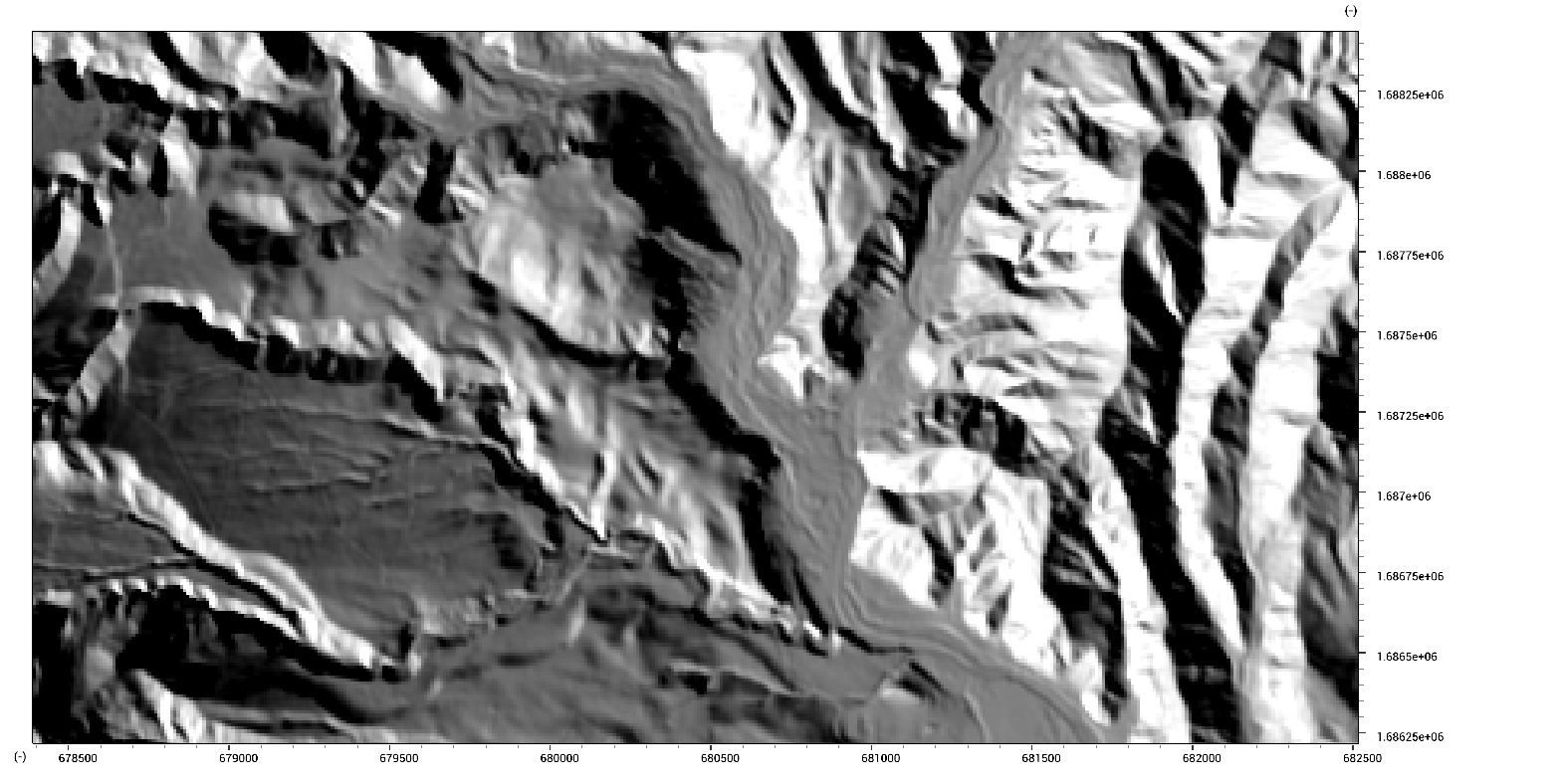Such a filter can be linearly blended according to slope to create a custom edge-preserving filter.

dem_lidar_wa2.map = min(1.0,max(0.0,1.0-Slope(dem_lidar.map)))*WindowAverage(dem_lidar.map,50) + min(1.0,max(0.0,Slope(dem_lidar.map)))*dem_lidar.map;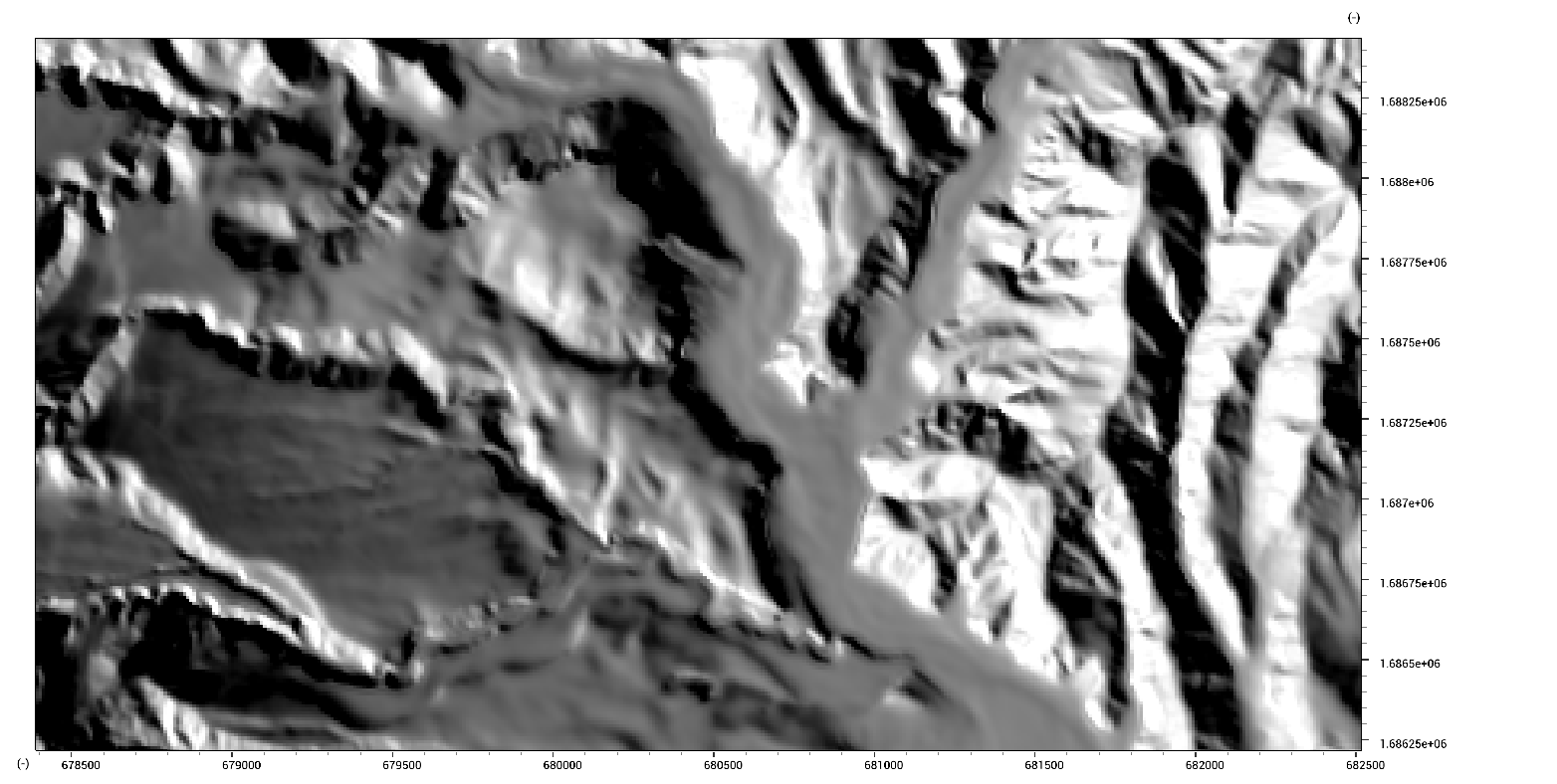The actual BilateralFilter, which is such an edge-preserving filter, works in a similar manner.

dem_lidar_wa.map = BilateralFilter(dem_lidar.map,10,5,5)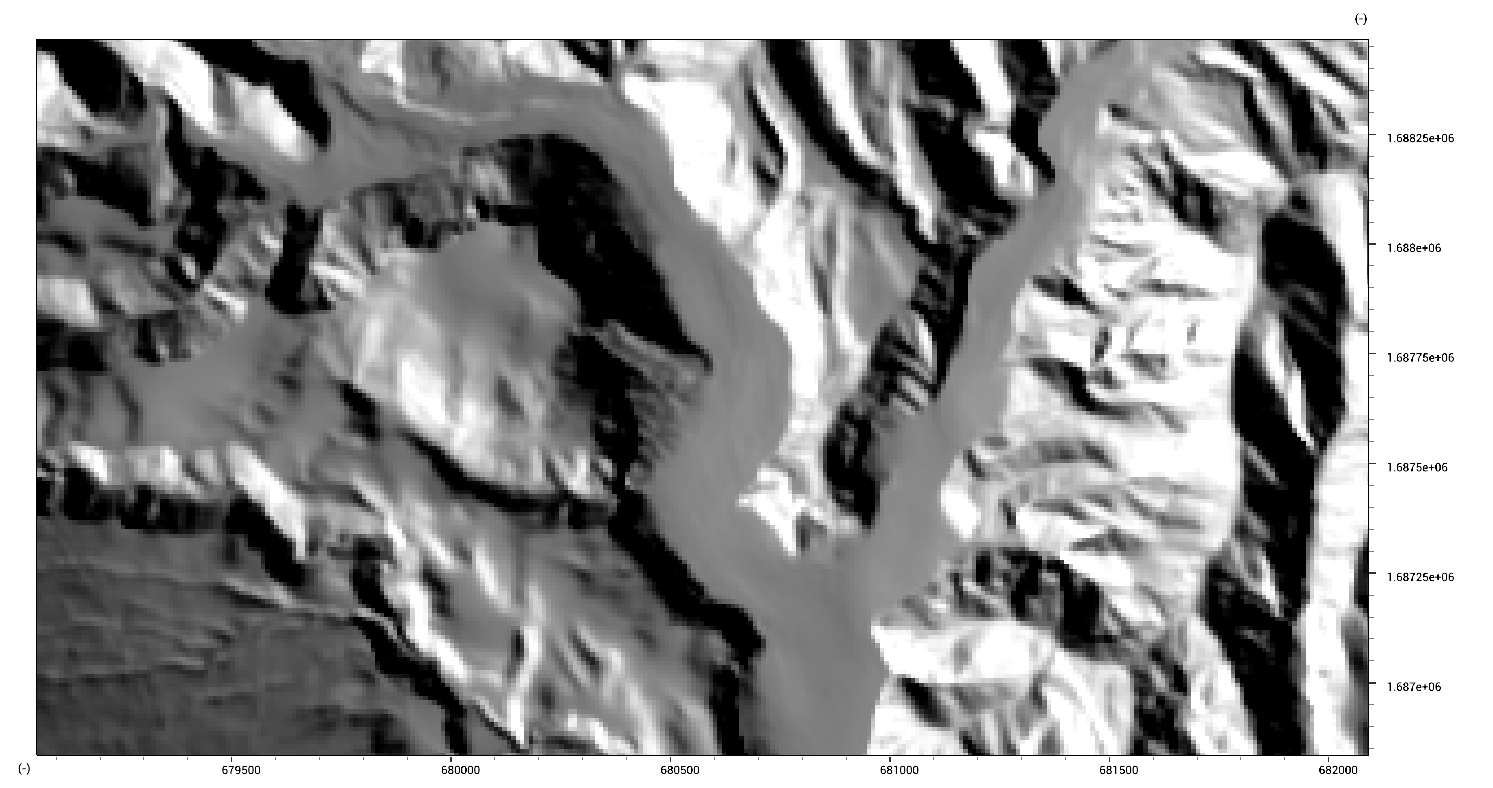Let us now add some random noise to the data by using the RandomMap function. This function gives each pixel a uniquely drawn random value between 0 and 1.

dem_lidar_noise.map = dem_lidar.map + MapIf(RandomMap(dem_lidar.map) >> 0.985,100.0* RandomMap(dem_lidar.map),0.0)We can isolate and remove such sparse errors by using noise filters. Two examples are the frost and lee noise filters.

dem_lidar_frost.map = NoiseFilterFrost(dem_lidar_noise.map,3,1)

dem_lidar_lee.map = NoiseFilterLee(dem_lidar_noise.map,3,1)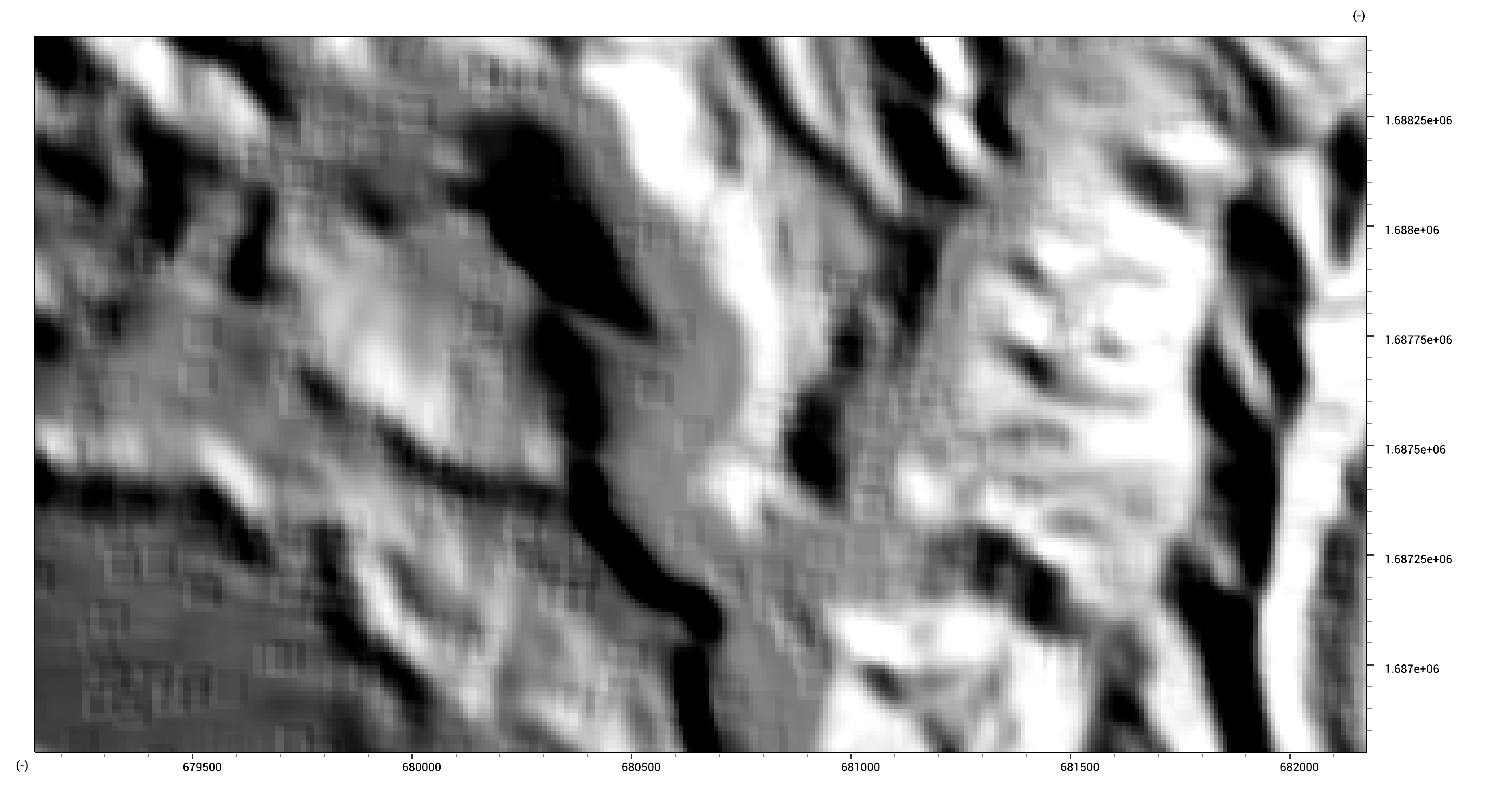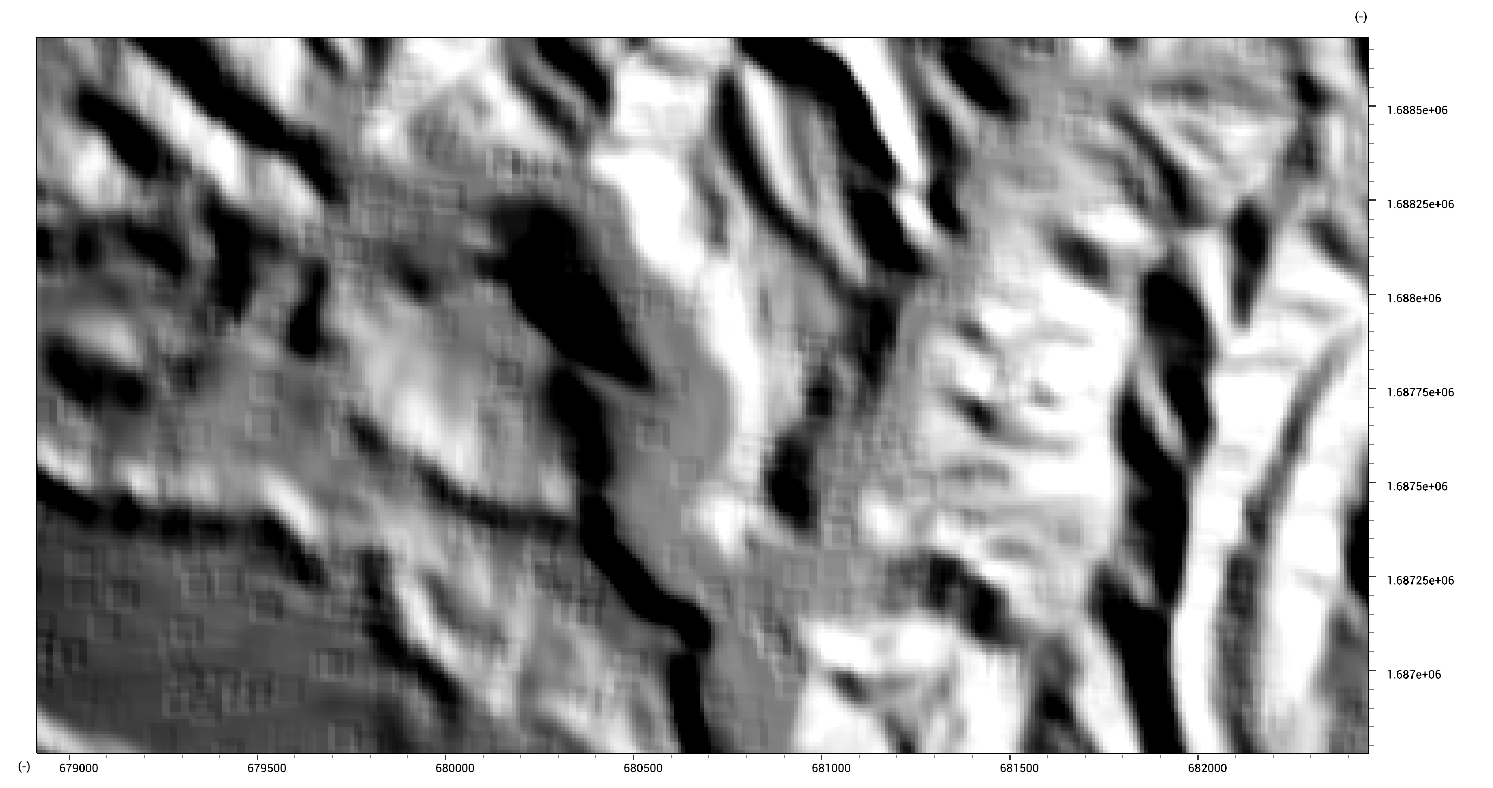Erosion and Dilution iteratively applies the erode and dilute operations. These operations are effectively similar to WindowMinimum(..,3cellsize) and WindowMaximum(..,3cellsize). On binary data, they shrink and grow regions.

dem_lidar_erode.map = Erode(dem_lidar_noise.map,3)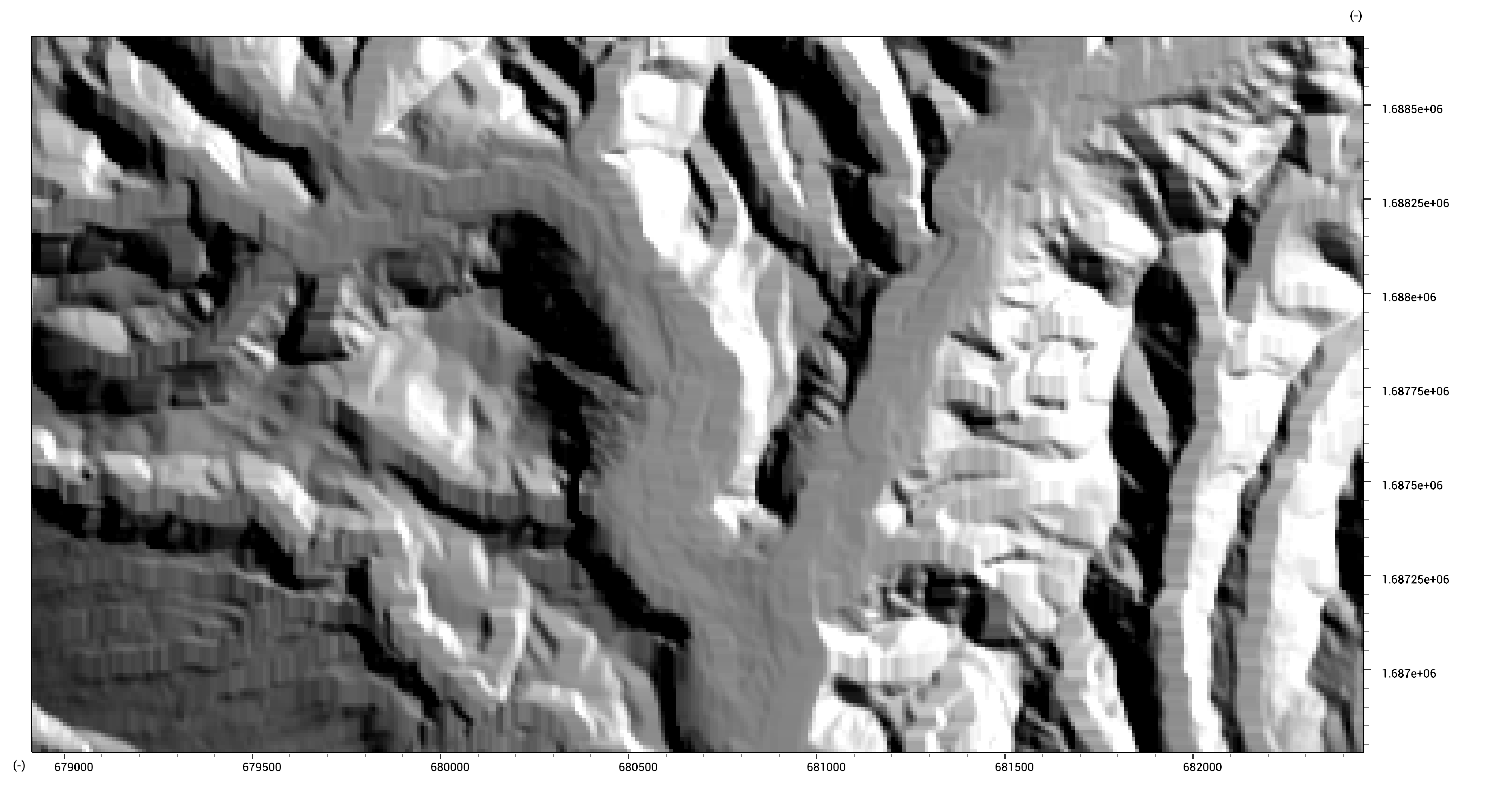Let us now apply a window-average filter to a dataset.

dem_lidar_av.map = WindowAverage(dem_lidar.map,60)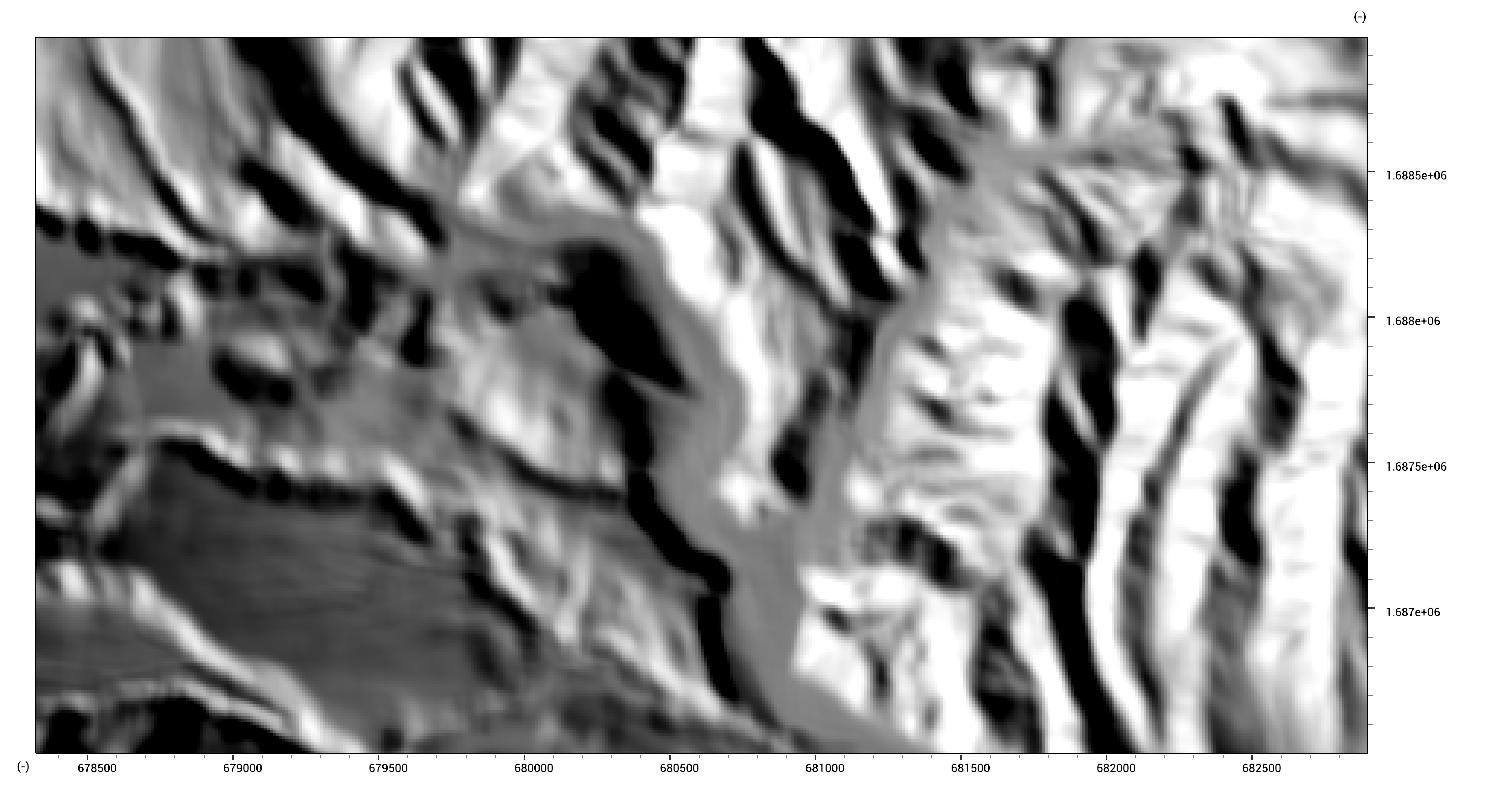Now, we can apply a deconvolution filter to attempt to undo the previous operation.

dem_lidar_deconvolve.map = RasterDeconvolve(dem_lidar.map,10,0.2)